# Riesz space

(diff) ← Older revision | Latest revision (diff) | Newer revision → (diff)

vector lattice

A real partially ordered vector space(cf. Partially ordered set; Vector space) in which

1) the vector space structure and the partial order are compatible. i.e. fromandfollows that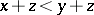and from,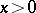,,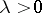follows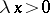;

2) for any two elements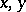there exists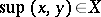. In particular, the supremum and infimum of any finite set exist.

In Soviet scientific literature Riesz spaces are usually called-lineals. Such spaces were first introduced by F. Riesz in 1928.

The space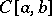of real continuous functions with the pointwise order is an example of a Riesz space. For any elementof a Riesz space one can define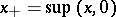,and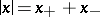. It turns out that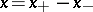. In Riesz spaces one can introduce two types of convergence of a sequence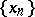. Order convergence,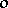-convergence: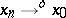if there exist a monotone increasing sequenceand a monotone decreasing sequence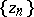such that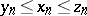and. Relative uniform convergence,-convergence: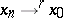if there exists an element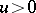such that for anythere exists an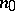such that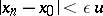for(-convergence is also called convergence with a regulator). The concepts of- and-convergence have many of the usual properties of convergence of numerical sequences and can be naturally generalized to nets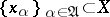.

A Riesz space is called Archimedean ifandfor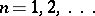imply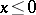. In Archimedean Riesz spaces,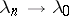andimply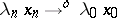(,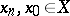), and-convergence implies-convergence.

How to Cite This Entry:
Riesz space. Encyclopedia of Mathematics. URL: http://encyclopediaofmath.org/index.php?title=Riesz_space&oldid=17299
This article was adapted from an original article by V.I. Sobolev (originator), which appeared in Encyclopedia of Mathematics - ISBN 1402006098. See original article